## Finite Field

A finite field is a Field with a finite Order (number of elements), also called a Galois Field. The order of a finite field is always a Prime or a Power of a Prime (Birkhoff and Mac Lane 1965). For each Prime Power, there exists exactly one (up to an Isomorphism) finite field GF(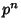), often written asin current usage. GF(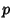) is called the Prime Field of order, and is the Field of Residue Classes modulo, where theelements are denoted 0, 1, ...,.in GF() means the same as. Note, however, that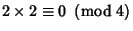in the Ring of residues modulo 4, so 2 has no reciprocal, and the Ring of residues modulo 4 is distinct from the finite field with four elements. Finite fields are therefore denoted GF(), instead of GF() for clarity.

The finite field GF(2) consists of elements 0 and 1 which satisfy the following addition and multiplication tables.0 1 0 0 1 1 1 00 1 0 0 0 1 0 1

If a subset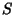of the elements of a finite field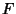satisfies the above Axioms with the same operators of, thenis called a Subfield. Finite fields are used extensively in the study of Error-Correcting Codes.

When, GF() can be represented as the Field of Equivalence Classes of Polynomials whose Coefficients belong to GF(). Any Irreducible Polynomial of degreeyields the same Field up to an Isomorphism. For example, for GF(), the modulus can be taken as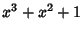,, or any other Irreducible Polynomial of degree 3. Using the modulus, the elements of GF()--written 0,,, ...--can be represented as Polynomials with degree less than 3. For instance,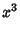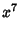Now consider the following table which contains several different representations of the elements of a finite field. The columns are the power, polynomial representation, triples of polynomial representation Coefficients (the vector representation), and the binary Integer corresponding to the vector representation (the regular representation).

 Power Polynomial Vector Regular 0 0 (000) 01 (001) 1(010) 2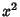(100) 4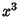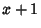(011) 3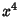(110) 6(111) 7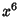(101) 5

The set of Polynomials in the second column is closed under Addition and Multiplication modulo, and these operations on the set satisfy the Axioms of finite field. This particular finite field is said to be an extension field of degree 3 of GF(2), written GF(), and the field GF(2) is called the base field of GF(). If an Irreducible Polynomial generates all elements in this way, it is called a Primitive Irreducible Polynomial. For any Prime or Prime Powerand any Positive Integer, there exists a Primitive Irreducible Polynomial of degreeover GF().

For any elementof GF(),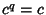, and for any Nonzero elementof GF(),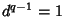. There is a smallest Positive Integersatisfying the sum condition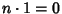in GF(), which is called the characteristic of the finite field GF(). The characteristic is a Prime Number for every finite field, and it is true that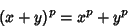over a finite field with characteristic.

References

Ball, W. W. R. and Coxeter, H. S. M. Mathematical Recreations and Essays, 13th ed. New York: Dover, pp. 73-75, 1987.

Birkhoff, G. and Mac Lane, S. A Survey of Modern Algebra, 3rd ed. New York: Macmillan, p. 413, 1965.

Dickson, L. E. History of the Theory of Numbers, Vol. 1: Divisibility and Primality. New York: Chelsea, p. viii, 1952.